Share

NCERT solutions for Class 10 Mathematics chapter 15 - Probability

Mathematics Textbook for Class 10

NCERT Mathematics Class 10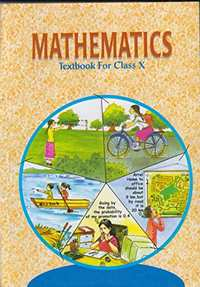Chapter 15: Probability

Ex. 15.10Ex. 15.20

Chapter 15: Probability Exercise 15.10 solutions [Pages 308 - 311]

Ex. 15.10 | Q 1.1 | Page 308

Complete the following statement

Probability of an event E + Probability of the event ‘not E’ = _______.

Ex. 15.10 | Q 1.2 | Page 308

Complete the following statement:

The probability of an event that cannot happen is _________. Such as event is called _________.

Ex. 15.10 | Q 1.3 | Page 308

Complete the following statement:

The probability of an event that is certain to happen is _________. Such as event is called ________

Ex. 15.10 | Q 1.4 | Page 308

Complete the following statement:

The sum of the probabilities of all the elementary events of an experiment is _________.

Ex. 15.10 | Q 1.5 | Page 308

Complete the following statement:

The probability of an event is greater than or equal to _______ and less than or equal to _______.

Ex. 15.10 | Q 2 | Page 308

Which of the following experiments have equally likely outcomes? Explain.

(1) A driver attempts to start a car. The car starts or does not start.

(2) A player attempts to shoot a basketball. She/he shoots or misses the shot.

(3i) A trial is made to answer a true-false question. The answer is right or wrong.

(4) A baby is born. It is a boy or a girl.

Ex. 15.10 | Q 3 | Page 308

Why is tossing a coin considered to be a fair way of deciding which team should get the ball at the beginning of a football game?

Ex. 15.10 | Q 4 | Page 308

Which of the following cannot be the probability of an event?

(A) 2/3

(B) –1.5

(C) 15%

(D) 0.7

Ex. 15.10 | Q 5 | Page 308

If P(E) = 0.05, what is the probability of ‘not E’?

Ex. 15.10 | Q 6.1 | Page 308

A bag contains lemon flavoured candies only. Malini takes out one candy without looking into the bag. What is the probability that she takes out an orange flavoured candy?

Ex. 15.10 | Q 6.2 | Page 308

A bag contains lemon flavoured candies only. Malini takes out one candy without looking into the bag. What is the probability that she takes out a lemon flavoured candy?

Ex. 15.10 | Q 7 | Page 308

It is given that in a group of 3 students, the probability of 2 students not having the same birthday is 0.992. What is the probability that the 2 students have the same birthday?

Ex. 15.10 | Q 8.1 | Page 308

A bag contains 3 red balls and 5 black balls. A ball is drawn at random from the bag. What is the probability that the ball drawn is not red?

Ex. 15.10 | Q 8.1 | Page 308

A bag contains 3 red balls and 5 black balls. A ball is drawn at random from the bag. What is the probability that the ball drawn is red?

Ex. 15.10 | Q 9 | Page 308

A box contains 5 red marbles, 8 white marbles and 4 green marbles. One marble is taken out of the box at random. What is the probability that the marble taken out will be (i) red? (ii) white? (iii) not green?

Ex. 15.10 | Q 10 | Page 309

A piggy bank contains hundred 50 p coins, fifty Rs 1 coins, twenty Rs 2 coins and ten Rs 5 coins. If it is equally likely that one of the coins will fall out when the bank is turned upside down, what is the probability that the coin

(i) Will be a 50 p coin?

(ii) Will not be a Rs.5 coin?

Ex. 15.10 | Q 11 | Page 309

Gopi buys a fish from a shop for his aquarium. The shopkeeper takes out one fish at random from a tank containing 5 male fish and 8 female fish (see Figure). What is the probability that the fish taken out is a male fish?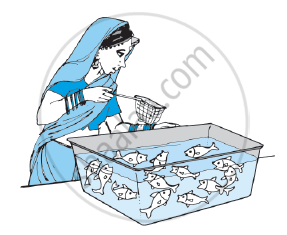Ex. 15.10 | Q 12 | Page 309

A game of chance consists of spinning an arrow which comes to rest pointing at one of the numbers 1, 2, 3, 4, 5, 6, 7, 8 (see the given figure), and these are equally likely outcomes. What is the probability that it will point at

(i) 8?

(ii) an odd number?

(iii) a number greater than 2?

(iv) a number less than 9?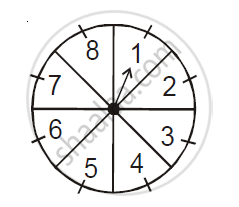Ex. 15.10 | Q 13.1 | Page 309

A die is thrown once. Find the probability of getting a prime number;

Ex. 15.10 | Q 13.2 | Page 309

A die is thrown once. Find the probability of getting a number lying between 2 and 6;

Ex. 15.10 | Q 13.3 | Page 309

A die is thrown once. Find the probability of getting

an odd number

Ex. 15.10 | Q 14.1 | Page 309

One card is drawn from a well-shuffled deck of 52 cards. Find the probability of getting a king of red colour.

Ex. 15.10 | Q 14.2 | Page 309

One card is drawn from a well-shuffled deck of 52 cards. Find the probability of getting a face card

Ex. 15.10 | Q 14.3 | Page 309

One card is drawn from a well-shuffled deck of 52 cards. Find the probability of getting a red face card.

Ex. 15.10 | Q 14.4 | Page 309

One card is drawn from a well-shuffled deck of 52 cards. Find the probability of getting the jack of hearts.

Ex. 15.10 | Q 14.5 | Page 309

One card is drawn from a well-shuffled deck of 52 cards. Find the probability of getting a spade.

Ex. 15.10 | Q 14.6 | Page 309

One card is drawn from a well-shuffled deck of 52 cards. Find the probability of getting the queen of diamonds.

Ex. 15.10 | Q 15.1 | Page 309

Five cards, the ten, jack, queen, king and ace of diamonds, are well-shuffled with their face downwards. One card is then picked up at random. What is the probability that the card is the queen?

Ex. 15.10 | Q 15.2 | Page 309

Five cards, the ten, jack, queen, king and ace of diamonds, are well-shuffled with their face downwards. One card is then picked up at random.

If the queen is drawn and put aside, what is the probability that the second card picked up is (a) an ace? (b) a queen?

Ex. 15.10 | Q 16 | Page 309

12 defective pens are accidentally mixed with 132 good ones. It is not possible to just look at a pen and tell whether or not it is defective. One pen is taken out at random from this lot. Determine the probability that the pen taken out is a good one.

Ex. 15.10 | Q 17 | Page 309

1) A lot of 20 bulbs contain 4 defective ones. One bulb is drawn at random from the lot. What is the probability that this bulb is defective?

2) Suppose the bulb drawn in (1) is not defective and is not replaced. Now one bulb is drawn at random from the rest. What is the probability that this bulb is not defective?

Ex. 15.10 | Q 18.1 | Page 309

A box contains 90 discs which are numbered from 1 to 90. If one disc is drawn at random from the box, find the probability that it bears a two-digit number

Ex. 15.10 | Q 18.2 | Page 309

A box contains 90 discs which are numbered from 1 to 90. If one disc is drawn at random from the box, find the probability that it bears a perfect square number

Ex. 15.10 | Q 18.3 | Page 309

A box contains 90 discs which are numbered from 1 to 90. If one disc is drawn at random from the box, find the probability that it bears a number divisible by 5.

Ex. 15.10 | Q 19 | Page 310

A child has a die whose six faces shows the letters as given below: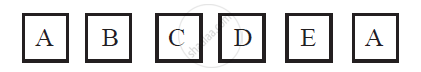The die is thrown once. What is the probability of getting (i) A? (ii) D?

Ex. 15.10 | Q 20 | Page 310

Suppose you drop a die at random on the rectangular region shown in the given figure. What is the probability that it will land inside the circle with diameter 1m?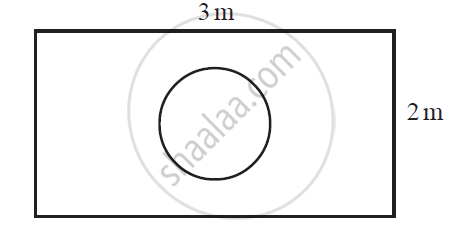Ex. 15.10 | Q 21.1 | Page 310

A lot consists of 144 ball pens of which 20 are defective and the others are good. Nuri will buy a pen if it is good, but will not buy if it is defective. The shopkeeper draws one pen at random and gives it to her. What is the probability that She will buy it?

Ex. 15.10 | Q 21.2 | Page 310

A lot consists of 144 ball pens of which 20 are defective and the others are good. Nuri will buy a pen if it is good, but will not buy if it is defective. The shopkeeper draws one pen at random and gives it to her. What is the probability that She will not buy it?

Ex. 15.10 | Q 22.1 | Page 310

Two dice, one blue and one grey, are thrown at the same time.

(i) Write down all the possible outcomes and complete the following table:

 Event :‘Sum on 2 dice’ 2 3 4 5 6 7 8 9 10 11 12 Probability 1/36 5/36 1/36
Ex. 15.10 | Q 22.2 | Page 310

A student argues that ‘there are 11 possible outcomes 2, 3, 4, 5, 6, 7, 8, 9, 10, 11 and 12. Therefore, each of them has a probability 1/11. Do you agree with this argument?

Ex. 15.10 | Q 23 | Page 310

A game consists of tossing a one rupee coin 3 times and noting its outcome each time. Hanif wins if all the tosses give the same result i.e., three heads or three tails, and loses otherwise. Calculate the probability that Hanif will lose the game.

Ex. 15.10 | Q 24 | Page 310

A die is thrown twice. What is the probability that

(i) 5 will not come up either time?

(ii) 5 will come up at least once?

[Hint: Throwinga die twice and throwing two dice simultaneously are treated as the same experiment].

Ex. 15.10 | Q 25.1 | Page 311

Which of the following arguments are correct and which are not correct? Give reasons for your answer.

If two coins are tossed simultaneously there are three possible outcomes, two heads, two tails or one of each. Therefore, for each of these outcomes, the probability is 1/3

Ex. 15.10 | Q 25.2 | Page 311

Which of the following arguments are correct and which are not correct? Give reasons for your answer.

If a die is thrown, there are two possible outcomes, an odd number or an even number. Therefore, the probability of getting an odd number is 1/2.

Chapter 15: Probability Exercise 15.20 solutions [Page 311]

Ex. 15.20 | Q 1 | Page 311

Two customers Shyam and Ekta are visiting a particular shop in the same week (Tuesday to Saturday). Each is equally likely to visit the shop on any day as on another day. What is the probability that both will visit the shop on

(i) the same day? (ii) consecutive days? (iii) different days?

Ex. 15.20 | Q 2 | Page 311

A die is numbered in such a way that its faces show the number 1, 2, 2, 3, 3, 6. It is thrown two times and the total score in two throws is noted. Complete the following table which gives a few values of the total score on the two throws: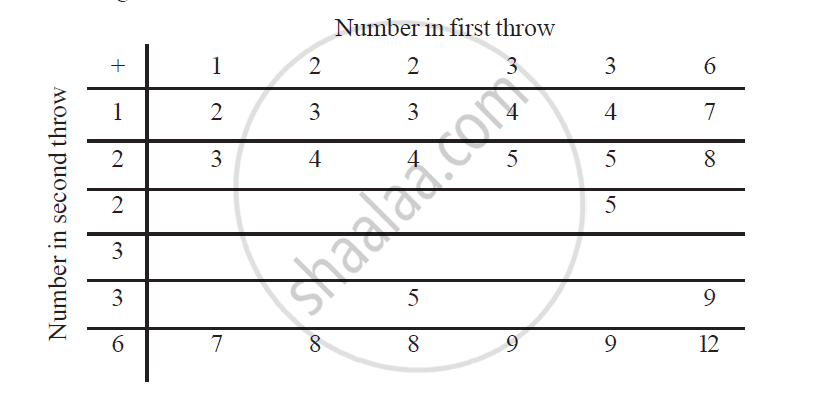What is the probability that the total score is

(i) even? (ii) 6? (iii) at least 6?

Ex. 15.20 | Q 3 | Page 311

A bag contains 5 red balls and some blue balls. If the probability of drawing a blue ball is double that of a red ball, determine the number of blue balls in the bag

Ex. 15.20 | Q 4 | Page 311

A box contains 12 balls out of which x are black. If one ball is drawn at random from the box, what is the probability that it will be a black ball?

If 6 more black balls are put in the box, the probability of drawing a black ball is now double of what it was before. Find x.

Chapter 15: Probability

Ex. 15.10Ex. 15.20

NCERT Mathematics Class 10NCERT solutions for Class 10 Mathematics chapter 15 - Probability

NCERT solutions for Class 10 Maths chapter 15 (Probability) include all questions with solution and detail explanation. This will clear students doubts about any question and improve application skills while preparing for board exams. The detailed, step-by-step solutions will help you understand the concepts better and clear your confusions, if any. Shaalaa.com has the CBSE Mathematics Textbook for Class 10 solutions in a manner that help students grasp basic concepts better and faster.

Further, we at Shaalaa.com are providing such solutions so that students can prepare for written exams. NCERT textbook solutions can be a core help for self-study and acts as a perfect self-help guidance for students.

Concepts covered in Class 10 Mathematics chapter 15 Probability are Sample Space, Concept Or Properties of Probability, Simple Problems on Single Events, Introduction to Probability, Probability - A Theoretical Approach, Probability Examples and Solutions.

Using NCERT Class 10 solutions Probability exercise by students are an easy way to prepare for the exams, as they involve solutions arranged chapter-wise also page wise. The questions involved in NCERT Solutions are important questions that can be asked in the final exam. Maximum students of CBSE Class 10 prefer NCERT Textbook Solutions to score more in exam.

Get the free view of chapter 15 Probability Class 10 extra questions for Maths and can use Shaalaa.com to keep it handy for your exam preparation

S